Next: Relative belief updating factor Up: The Bayesian way out: Previous: The Bayesian way out:

## Models for Galactic sources of gravitational waves

Having seen that the analysis has to be based on models for the emission of g.w.'s, let us focus on some popular models within Galaxy. This limitation is due to the sensitivity of the ROG detectors. The models taken into account are: emission only from sources concentrated in the Galactic Center (GC); emission from sources uniformly distributed over the Galactic Disk (GD); emission from sources distributed as the known visible mass of Galaxy (GMD). In addition we have also included the non-Galactic model of sources isotropically distributed around Earth (ISO). In this context, this latter model can just be seen as an academic example to give a feeling of what the analysis method would produce in such a scenario.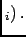The pattern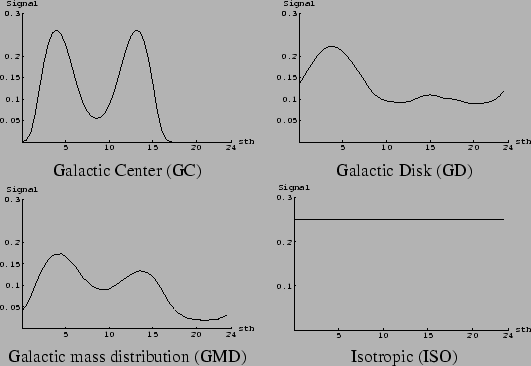of the two detectors Explorer and Nautilus to signals due to these models are shown in Fig. 2. These patterns are all given as a function of the sidereal time.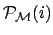depends on the space and energy distributions of g.w. sources described by a model, taking also into account the variation of the detector efficiency with the sidereal time, due to the varying orientation of the antennae respect to the sources. It depends also on the (unknown) energies of g.w. signals, on the noise, and on the coordinates (latitude,longitude,azimuth) of the detectors on Earth. Simply speaking, for various models of the space distribution of sources of g.w., we expect different responses from the coincidence experiment, i.e. different antenna patterns', seen as a function of the sidereal time.

The calculation ofdepends on the expected g.w. energy and some assumptions are needed. The signal's amplitudeis unknown, and thus we have evaluated the patterns by integrating over a uniform distribution ofvalues, ranging - on Earth - fromup to. Different reasonings may be done here, leading to different choices for the amplitudes range and for the distributions of the signals. We have done the simplest choice, supposing that we do not know anything on the signals, but the fact that no signals have been observed at the detectors with amplitude greater than. The lowest limit has been chosen considering the fact that the detector's efficiencies belowis very small (less than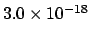). Note that the consideredvalues are based on standard' assumptions about the g.w. energy release in cryogenic bars. The Galactic Disk (GD) model has been constructed considering g.w. sources uniformly distributed over the Galactic plane, which means a distribution of sources which is not uniform around the Earth, given the fact that the Earth is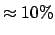kpc from the Center of Galaxy, which is the center of the disk (whose radius is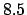kpc). The GMD distribution, taking into account the mass distribution in Galaxy, is much more interesting than the GD model. In fact we do not expect a uniform distribution of the sources over the GD, but a distribution which is concentrated near the GC .Next: Relative belief updating factor Up: The Bayesian way out: Previous: The Bayesian way out:
Giulio D'Agostini 2005-01-09